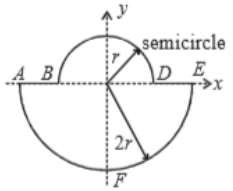A uniform thin rod is bent in the form of closed loop ABCDEFA as shown in the figure. The ratio of inertia of the loop about x-axis to that about y-axis is:1.  >1

2.  <1

3.  =1

4.  =1/2

Concept Videos :-

#10 | Moment of Inertia (MI): Introduction
#11 | MI: Discreet Particles
#12 | MI: Continuous Bodies_1
#13 | MI: Questions on Continuous Bodies
#14 | MI: Continuous Bodies_2
#15 | Theorems of Moment of Inertia
#16 | Questions on Theorems of MI
#17 | Set 1: Previous Year Problems

Concept Questions :-

Moment of inertia

Difficulty Level:

• 25%
• 45%
• 20%
• 11%